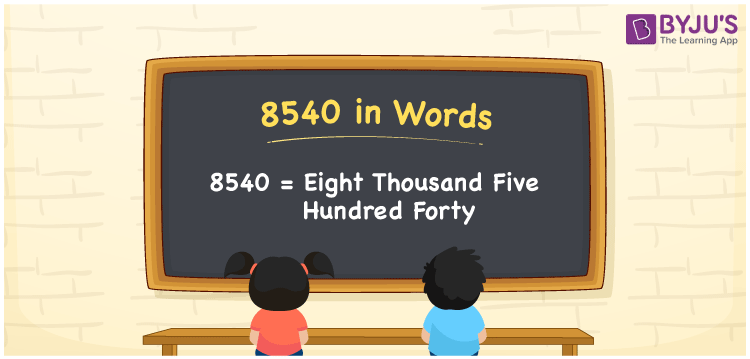# 8540 in words

8540 in words is written as Eight Thousand Five Hundred and Forty. 8540 represents the count or value. The article on Counting Numbers can give you an idea about count or counting. The number 8540 is used in expressions that relate to money, distance, length and others. Let us consider an example for 8540. ”Can I buy a golden pendant for Eight Thousand Five Hundred and Forty rupees?”

 8540 in words Eight Thousand Five Hundred and Forty Eight Thousand Five Hundred and Forty in Numbers 8540

## 8540 in English Words## How to Write 8540 in Words?

We can convert 8540 to words using a place value chart. The number 8540 has 4 digits, so let’s make a chart that shows the place value up to 4 digits.

 Thousands Hundreds Tens Ones 8 5 4 0

Thus, we can write the expanded form as:

8 × Thousand + 5 × Hundred + 4 × Ten + 0 × One

= 8 × 1000 + 5 × 100 + 4 × 10 + 0 × 1

= 8540

= Eight Thousand Five Hundred and Forty.

8540 is the natural number that is succeeded by 8539 and preceded by 8541.

8540 in words – Eight Thousand Five Hundred and Forty.

Is 8540 an odd number? – No.

Is 8540 an even number? – Yes.

Is 8540 a perfect square number? – No.

Is 8540 a perfect cube number? – No.

Is 8540 a prime number? – No.

Is 8540 a composite number? – Yes.

## Solved Example

1. Write the number 8540 in expanded form

Solution: 8 × 1000 + 5 × 100 + 4 × 10 + 0 × 1

We can write 8540 = 8000 + 500 + 40 + 0

= 8 × 1000 + 5 × 100 + 4 × 10 + 0 × 1

## Frequently Asked Questions on 8540 in words

Q1

### How to write 8540 in words?

8540 in words is written as Eight Thousand Five Hundred and Forty.
Q2

### Is 8540 a perfect square number?

No. 8540 is not a perfect square number.
Q3

### Is 8540 a prime number?

No. 8540 is not a prime number.